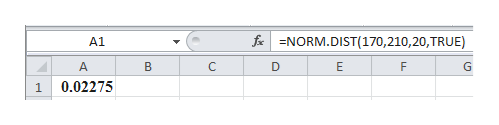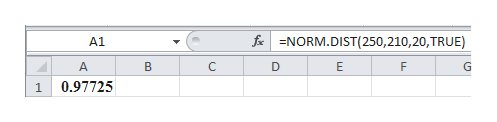Suppose that for a given animal, the length of pregnancy from conception to birth varies according to a distribution that is approximately normal with mean210 days and standard deviation 20 days. We can expect that about __ percent of all completed pregnancies for this animal are between 170 and 250 days.(answer must be answered in percent form)

Question

Suppose that for a given animal, the length of pregnancy from conception to birth varies according to a distribution that is approximately normal with mean210 days and standard deviation 20 days. We can expect that about __ percent of all completed pregnancies for this animal are between 170 and 250 days.

(answer must be answered in percent form)

Step 1

It is given that mean and standard deviations of normal distribution are 210 days and 20 days respectively.

The probability that all completed pregnancies less than 170 can be calculated by entering the formula ‘=NORM.DIST(170,210,20,TRUE)’ in EXCEL software.help_outlineImage TranscriptioncloseNORM.DIST(170, 210, 20, TRUE) f A1 A B D E G 0.02275 1 fullscreen
Step 2

The probability that all completed pregnancies less than 250 can be calculated by entering the formula ‘=NORM.DIST(250,210,20,TRUE)’ in EXCEL software.help_outlineImage Transcriptionclosef NORM.DIST(250, 210, 20, TRUE) A1 A B D G 0.97725 1 fullscreen
Step 3

The probability that all completed pregnancies bet...

Want to see the full answer?

See Solution

Want to see this answer and more?

Our solutions are written by experts, many with advanced degrees, and available 24/7

See Solution
Tagged in

Random Variables